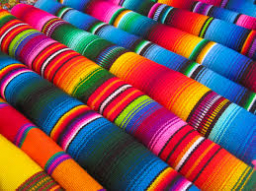# Math:

Math: solve each problem.
1. for the school's intramurals, a group of students prepared 23 1/2 liters of lemonade to sell. They had 3 5/8 liters left over. How many liters of lemonade were sold?
2. My mother bought 3 3/4 kg of beef, 23/5 kg of pork, and 5 1/2 kg of chicken. How many kilograms of meat did she buy?
3. Mr. De Guzman bought 7 1/2 kg of meat. He used 2 3/4 kilograms of afritada, 3 1/8 kg for menudo, and the rest for pochero. How many kilograms of meat did he use for pochero?
4. How much greater is the difference of 8 3/4 and 4 1/2 than the sum of 1 2/3 and 1 5/8?
5. Karen's father has 18 3/8 m nylon strings. He gave 10 5/9 m of it for his friend's fishing rod. About how long is the nylon string that is left?

a =  19 78 = 159/8
b =  19 78 = 159/8 kg
c =  1 58 = 13/8 kg
d =  23/24
e =  7 5972 = 563/72 m

### Step-by-step explanation:Did you find an error or inaccuracy? Feel free to write us. Thank you!

Tips for related online calculators
Need help calculating sum, simplifying, or multiplying fractions? Try our fraction calculator.
Need help with mixed numbers? Try our mixed-number calculator.
Do you want to convert length units?
Tip: Our volume units converter will help you convert volume units.